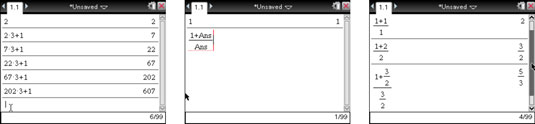##### TI-Nspire For Dummies, 2nd EditionEach time you perform a calculation, the result is stored to TI-Nspire’s local memory as the Ans variable. As a result, you can quickly access this stored variable and use it in subsequent calculations.

Consider, for example, that you want to teach students how to evaluate an algebraic expression, such as 2x2 + 1 for x = –8. This problem can be easily evaluated by entering the entire expression once on the entry line.

However, perhaps you want to take students through the process, step by step, to place additional emphasis on the order of operations. Here’s how the Last Answer feature can accomplish this task:

1. Type –8 on the entry line and press [ENTER].

This stores the number –8 to the last answer Ans variable.

2. Press the [x2] key.

This action automatically pastes the Ans variable to the entry line and raises it to the second power.

3. Press [ENTER] to square the last answer.

This stores the number 64 to the variable Ans.

4. Press [x] to multiply the last answer by 2.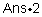5. Press [ENTER] to evaluate this expression.

This stores the number 128 to the Ans variable.

6. Press [–] to paste the expression Ans1 to the entry line. Press [ENTER] to find that the value of 2x2 – 1 for x = –8 is equal to 127.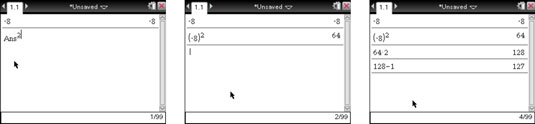Notice that whenever the Ans variable appears in the entry line, it is replaced with a numeric value after you press [ENTER].

## Use the TI-Nspire last answer variable in expressions

The TI-Nspire last answer variable can also be accessed by pressing [CTRL[[(-)], which is very helpful if you want to take your last answer from a calculation and use it one or more times in a subsequent calculation.

For example, consider a cylinder of known volume and height (volume = 50 and height = 10); you want to find the volume of a sphere with the same radius. Using the formula for volume, you can solve for the radius, as shown in the first screen. The last answer from this calculation, accessed by pressing [CTRL[[(-)], is used as the radius to find the volume of the sphere.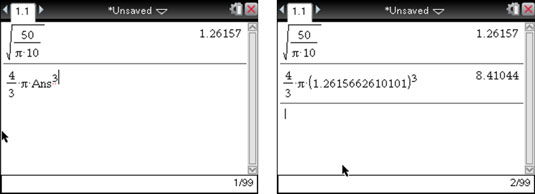## Use the TI-Nspire last answer variable to generate sequences

You can also use the last answer variable on the TI-Nspire to generate a sequence, such as 2, 5, 14, 41, . . . Each term of this sequence is found by multiplying the previous value by 3 and subtracting 1. Follow these steps to generate successive terms of this sequence:

1. Press [ENTER].

This action stores the first value of the sequence, 2, to the last answer variable.

2. Press [x][–] to paste the expression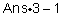to the entry line.

3. Press [ENTER] to evaluate this expression.

Notice that the expressionis replaced by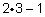indicating that the value of Ans in the previous step is equal to 2.

4. Continue to press [ENTER] to generate additional terms of this sequence. The second and third screens show how to generate a more complicated sequence of numbers. Perhaps you recognize the numerators and denominators of each fractional answer as terms from the Fibonacci sequence.